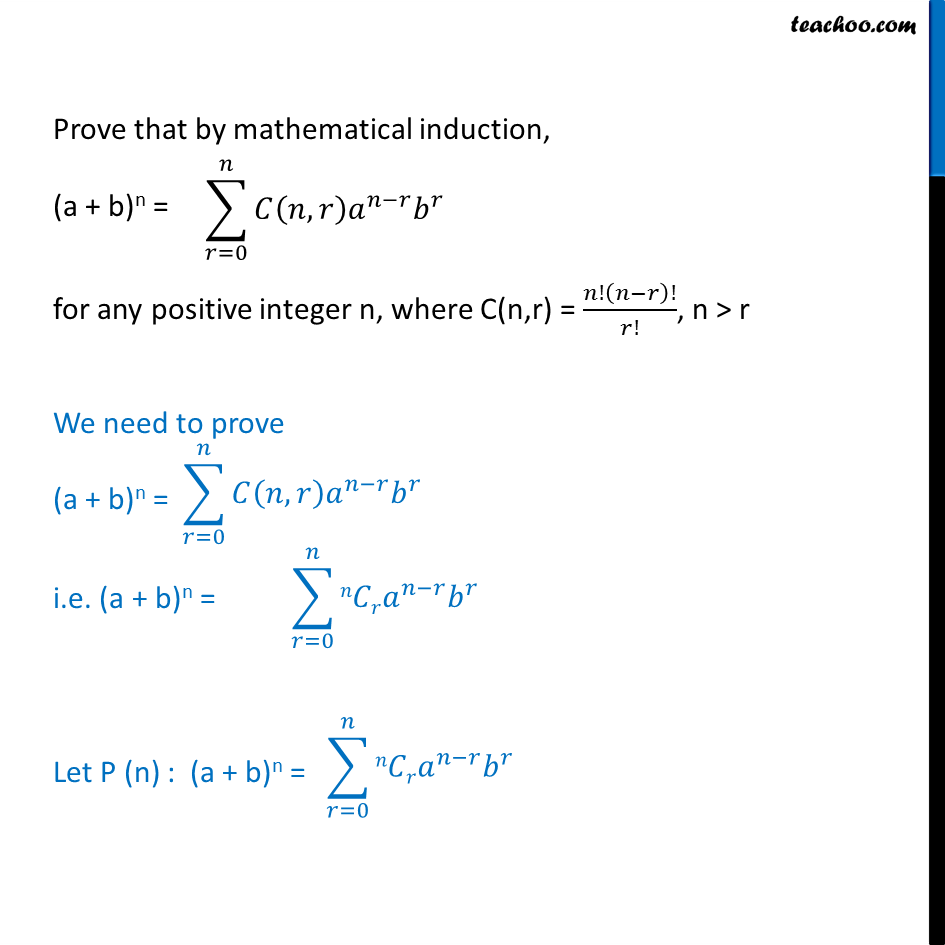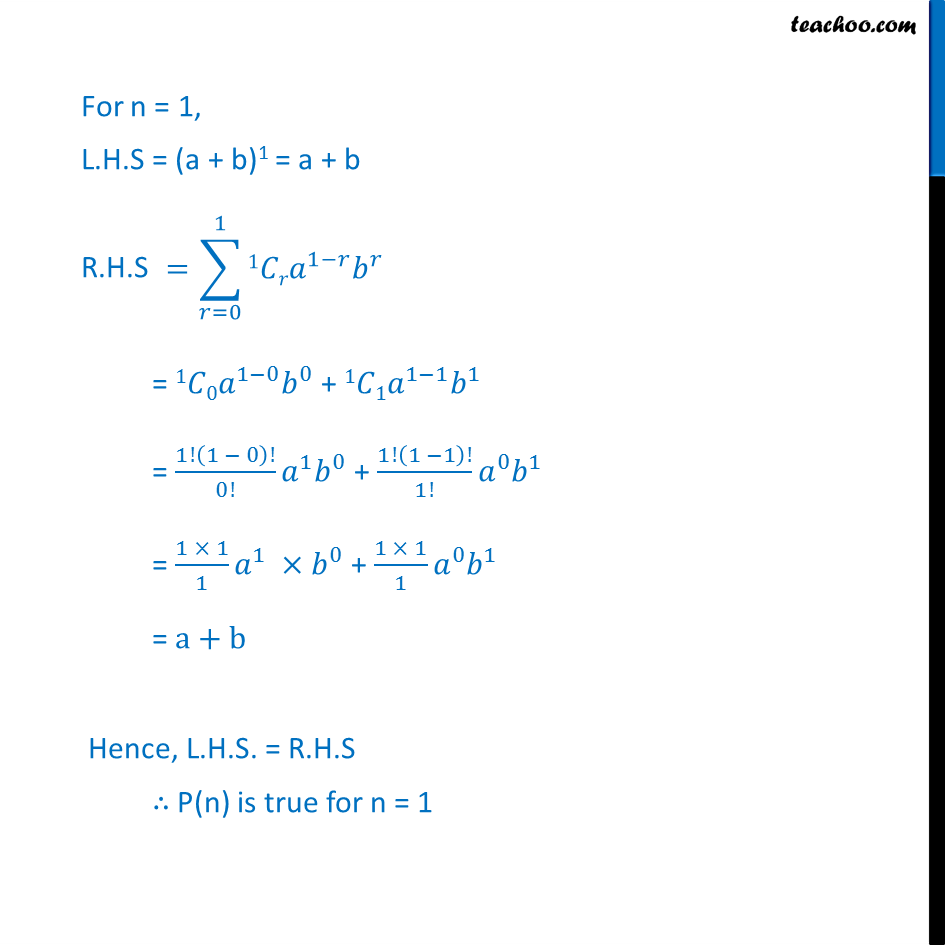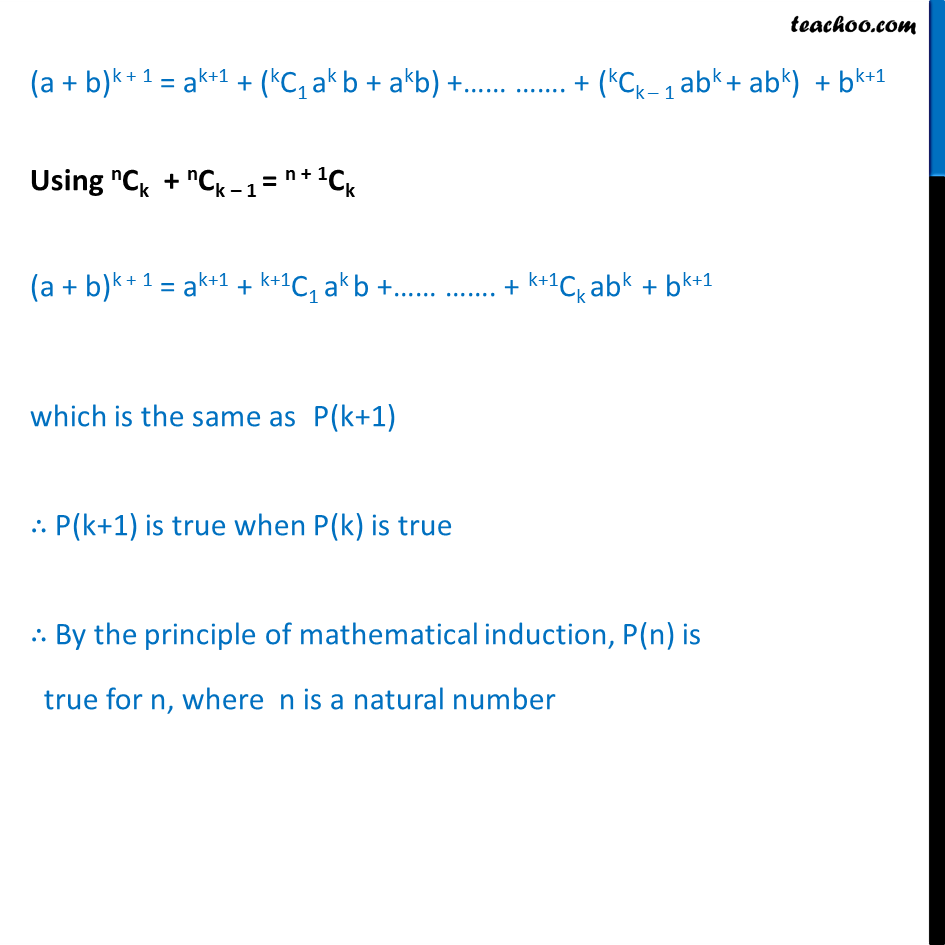Mathematical Induction
Concept wiseLearn in your speed, with individual attention - Teachoo Maths 1-on-1 Class

### Transcript

Prove binomial theorem by mathematical induction. i.e. Prove that by mathematical induction, (a + b)^n = 𝐶(𝑛,𝑟) 𝑎^(𝑛−𝑟) 𝑏^𝑟 for any positive integer n, where C(n,r) = 𝑛!(𝑛−𝑟)!/𝑟!, n > r We need to prove (a + b)n = ∑_(𝑟=0)^𝑛▒〖𝐶(𝑛,𝑟) 𝑎^(𝑛−𝑟) 𝑏^𝑟 〗 i.e. (a + b)n = ∑_(𝑟=0)^𝑛▒〖𝑛𝐶𝑟𝑎^(𝑛−𝑟) 𝑏^𝑟 〗 Let P (n) : (a + b)n = ∑_(𝑟=0)^𝑛▒〖𝑛𝐶𝑟𝑎^(𝑛−𝑟) 𝑏^𝑟 〗 For n = 1, L.H.S = (a + b)1 = a + b R.H.S = ∑_(𝑟=0)^1▒〖1𝐶𝑟𝑎^(1−𝑟) 𝑏^𝑟 〗 = 1𝐶0𝑎^(1−0) 𝑏^0 + 1𝐶1𝑎^(1−1) 𝑏^1 = 1!(1 − 0)!/0! 𝑎^1 𝑏^0 + 1!(1 −1)!/1! 𝑎^0 𝑏^1 = (1 × 1)/1 𝑎^1 ×𝑏^0 + (1 × 1)/1 𝑎^0 𝑏^1 = a+b Hence, L.H.S. = R.H.S ∴ P(n) is true for n = 1 Assume that P(k) is true (a + b)k = (a + b)k = kC0 ak b0 + kC1 ak – 1 b1 + …. …. + kCk – 1 a1 bk – 1 + kCk a0 bk (a + b)k = ak + kC1 ak – 1 b + …. …. + kCk – 1 a1 bk – 1 + bk We will prove that P(k + 1) is true. (a + b)k + 1 =∑_(𝑟=0)^(𝑘+1)▒〖 𝐶𝑟𝑎^(𝑘+1− 𝑟) 𝑏^𝑟 〗 (a + b)k = k+1C0 ak+1 b0 + k+1C1 ak + 1 – 1 b1 …. …. …….…. + k+1Ck – 1 a1 bk+ 1 – 1 + k+1Ck a0 bk+1 (a + b)k = ak+1 + k+1C1 ak b + …. …. + k+1Ck – 1 a bk + bk+1 We have to prove P(k+1) from P(k) i.e. (2) from (1) From (1) (a + b)k = ak + kC1 ak – 1 b + …. …. + kCk – 1 a1 bk – 1 + bk Multiplying (a + b) both sides, (a + b)k (a + b) = (a + b) (ak + kC1 ak – 1 b + …. …. + kCk – 1 a1 bk – 1 + bk ) (a + b)k + 1 = a (ak + kC1 ak – 1 b + …. …. + kCk – 1 a1 bk – 1 + bk ) + b (ak + kC1 ak – 1 b + …. …. + kCk – 1 a1 bk – 1 + bk ) (a + b)k + 1 = ak+1 + kC1 ak b + …. …. + kCk – 1 a2 bk – 1 + abk + bak + kC1 ak – 1 b2 + …. …. + kCk – 1 abk + bk+1 (a + b)k + 1 = ak+1 + (kC1 ak b + akb) +…… ……. + (kCk – 1 abk + abk) + bk+1 Using nCk + nCk – 1 = n + 1Ck (a + b)k + 1 = ak+1 + k+1C1 ak b +…… ……. + k+1Ck abk + bk+1 which is the same as P(k+1) ∴ P(k+1) is true when P(k) is true ∴ By the principle of mathematical induction, P(n) is true for n, where n is a natural number